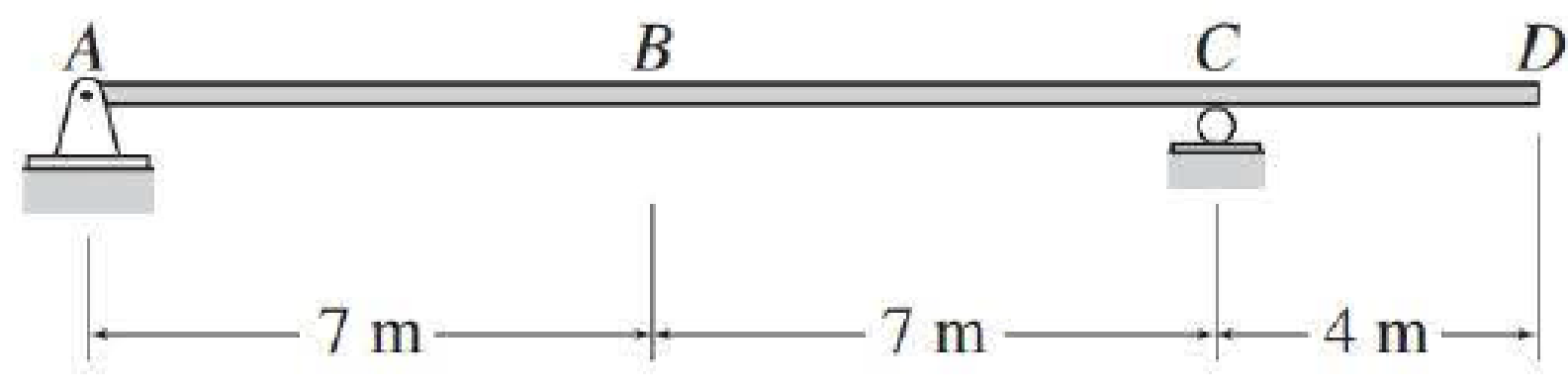# Draw the influence lines for the vertical reactions at support A and C and the shear and bending moment at point B of the beam shown in Fig. P8.6. FIG. P8.6, P8.61

#### Solutions

Chapter
Section
Chapter 8, Problem 6P
Textbook Problem
104 views

## Draw the influence lines for the vertical reactions at support A and C and the shear and bending moment at point B of the beam shown in Fig. P8.6.FIG. P8.6, P8.61

To determine

Draw the influence lines for the vertical reactions at supports A and C.

Draw the influence lines for the shear and bending moment at point B.

### Explanation of Solution

Calculation:

Apply a 1 kN unit moving load at a distance of x from left end A.

Sketch the free body diagram of beam as shown in Figure 1.

Refer Figure 1.

Find the equation of support reaction (Cy) at C using equilibrium equation:

Consider moment equilibrium at point A.

Consider clockwise moment as positive and anticlockwise moment as negative

Sum of moment at point A is zero.

ΣMA=0Cy(14)1(x)=014Cy=xCy=x14 (1)

Find the equation of support reaction (Ay) at A using equilibrium equation:

Apply vertical equilibrium equation of forces.

Consider upward force as positive (+) and downward force as negative ().

Ay+Cy=1

Substitute x14 for Cy.

Ay+x14=1Ay=1x14 (2)

Consider Equation (2).

Find the value of influence line ordinate of reaction Ay at support A.

Substitute 0 for x in Equation (2).

Ay=1014=1kN/kN

Similarly calculate the influence line ordinate of reaction Ay for different value of x and summarize the result in Table 1.

 x (m) Ay (kN/kN) 0 1 14 0 18 −27

Draw the influence line diagram for the vertical reactions at support A using Table 1 as shown in Figure 2.

Consider Equation (1).

Find the influence line ordinate of reaction Cy at support C.

Substitute 0 for x in Equation (1).

Cy=014=0

Similarly calculate the influence line ordinate of reaction Cy for different value of x and summarize the result in Table 2.

 x (m) Cy (kN/kN) 0 0 14 1 18 97

Draw the influence line diagram for the vertical reactions at support C using Table 2 as shown in Figure 3.

Find the equation of shear force at B of portion AB (0x<7m).

Sketch the free body diagram of the section AB as shown in Figure 4.

Refer Figure 4.

Apply equilibrium equation of forces.

Consider upward force as positive (+) and downward force as negative ().

ΣFy=0

AySB1=0SB=Ay1

Substitute 1x14 for Ay.

SB=(1x14)1=x14

Find the equation of shear force at B of portion BD (7m<x18m).

Sketch the free body diagram of the section BD as shown in Figure 5.

Refer Figure 5.

Apply equilibrium equation of forces.

Consider upward force as positive (+) and downward force as negative ()

### Still sussing out bartleby?

Check out a sample textbook solution.

See a sample solution

#### The Solution to Your Study Problems

Bartleby provides explanations to thousands of textbook problems written by our experts, many with advanced degrees!

Get Started

Find more solutions based on key concepts
Find the equation that best fits the following set of data points. Compare the actual and predicted y values. P...

Engineering Fundamentals: An Introduction to Engineering (MindTap Course List)

What are the corresponding centerline and included angles to 5/8" TPF?

Precision Machining Technology (MindTap Course List)

Why is an intercooler used on turbocharged and supercharged engines?

Automotive Technology: A Systems Approach (MindTap Course List)

Describe data and process modeling concepts and tools.

Systems Analysis and Design (Shelly Cashman Series) (MindTap Course List)

Compressed files are sometimes called _____ files.

Enhanced Discovering Computers 2017 (Shelly Cashman Series) (MindTap Course List)

If your motherboard supports ECC DDR3 memory, can you substitute non-ECC DDR3 memory?

A+ Guide to Hardware (Standalone Book) (MindTap Course List)